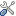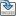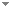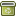# Programming Planck units from a virtual electron; a Simulation Hypothesis (summary)

Eur. Phys. J. Plus 133:278 (2018)

 Authors Abstract The Simulation Hypothesis proposes that all of reality, including the earth and the universe, is in fact an artificial simulation, analogous to a computer simulation, and as such our reality is an illusion. In this essay I describe a method for programming mass, length, time and charge (MLTA) as geometrical objects derived from the formula for a virtual electron; $f_e = 4\pi^2r^3$ ($r = 2^6 3 \pi^2 \alpha \Omega^5$) where the fine structure constant $\alpha$ = 137.03599... and $\Omega$ = 2.00713494... are mathematical constants and the MLTA geometries are; M = (1), T = ($2\pi$), L = ($2\pi^2\Omega^2$), A = ($4\pi \Omega)^3/\alpha$. As objects they are independent of any set of units and also of any numbering system, terrestrial or alien. As the geometries are interrelated according to $f_e$, we can replace designations such as ($kg, m, s, A$) with a rule set; mass = $u^{15}$, length = $u^{-13}$, time = $u^{-30}$, ampere = $u^{3}$. The formula $f_e$ is unit-less ($u^0$) and combines these geometries in the following ratio M$^9$T$^{11}$/L$^{15}$ and (AL)$^3$/T, as such these ratio are unit-less. To translate MLTA to their respective SI Planck units requires an additional 2 unit-dependent scalars. We may thereby derive the CODATA 2014 physical constants via the 2 (fixed) mathematical constants ($\alpha, \Omega$), 2 dimensioned scalars and the rule set $u$. As all constants can be defined geometrically, the least precise constants ($G, h, e, m_e, k_B$...) can also be solved via the most precise ($c, \mu_0, R_\infty, \alpha$), numerical precision then limited by the precision of the fine structure constant $\alpha$. Keywords black hole electron  Mathematical Universe  fine structure constant alpha  Planck units  Simulation Hypothesis  Simulated Universe  Planck momentum  magnetic monopole  mathematical electron  Virtual electron Categories (categorize this paper) OptionsEdit this recordMark as duplicateExport citationFind it on ScholarRequest removal from indexRevision history

PhilArchive copy

Setup an account with your affiliations in order to access resources via your University's proxy server
Configure custom proxy (use this if your affiliation does not provide a proxy)

## References found in this work BETA

The Mathematical Universe.Max Tegmark - 2007 - Foundations of Physics 38 (2):101-150.
.Nick Bostrom & Julian Savulescu - 2009 - Oxford University Press.

## Citations of this work BETA

No citations found.

## Similar books and articles

Fundamental Nature of the Fine-Structure Constant.Michael A. Sherbon - 2014 - International Journal of Physical Research 2 (1):1-9.
Quintessential Nature of the Fine-Structure Constant.Michael A. Sherbon - 2015 - Global Journal of Science Frontier Research: A Physics and Space Science 15 (4):23-26.
Fine Tuning Explained? Multiverses and Cellular Automata.Francisco José Soler Gil & Manuel Alfonseca - 2013 - Journal for General Philosophy of Science / Zeitschrift für Allgemeine Wissenschaftstheorie 44 (1):153-172.
A New Foundation for Physics.Jim Bourassa & David Thomson - 2006 - Infinite Energy Magazine (69):34.